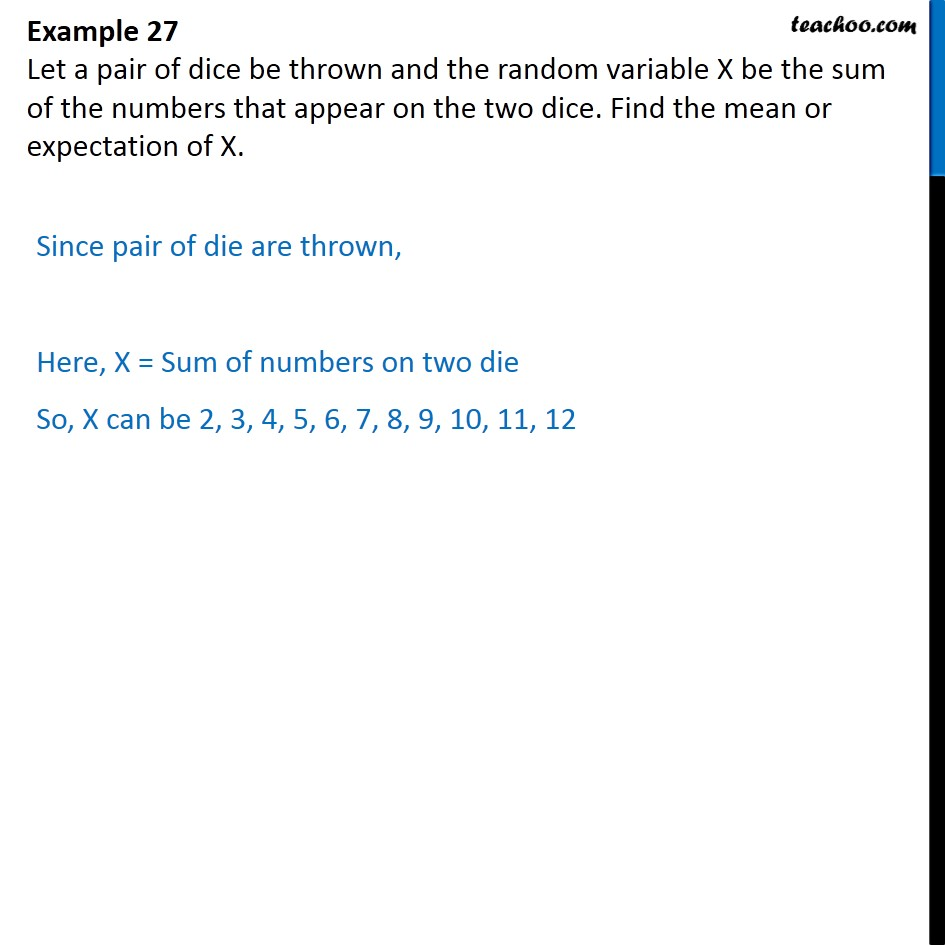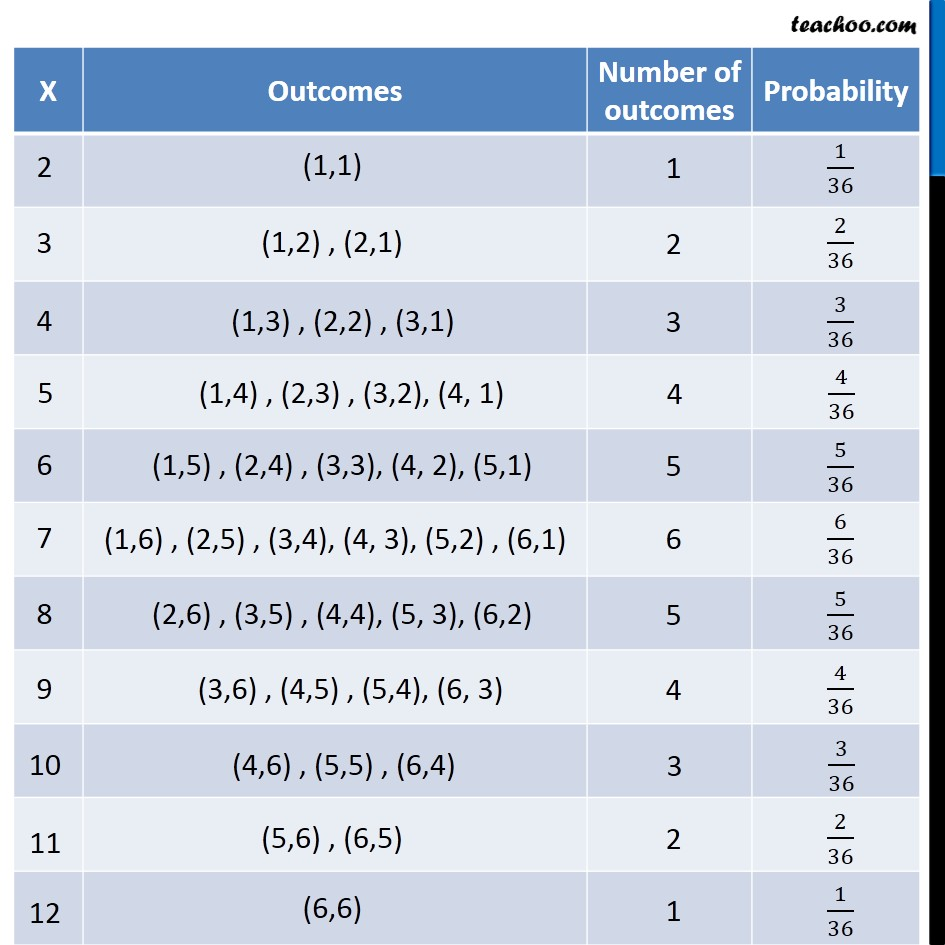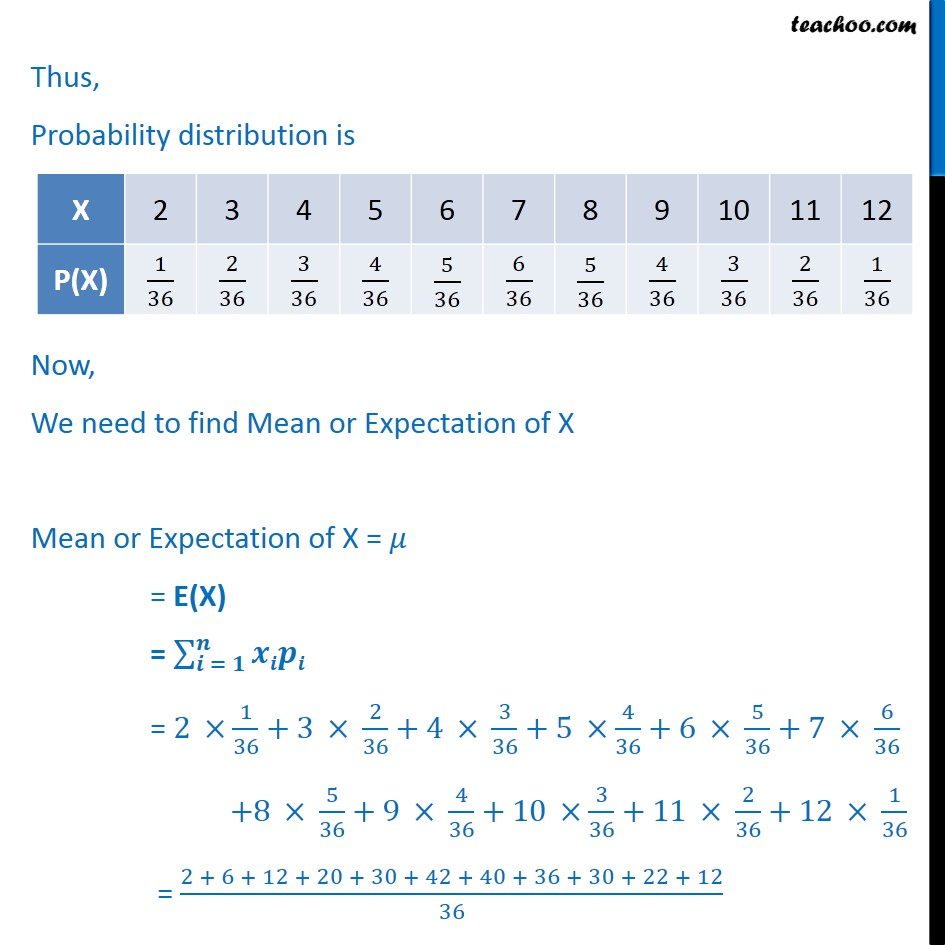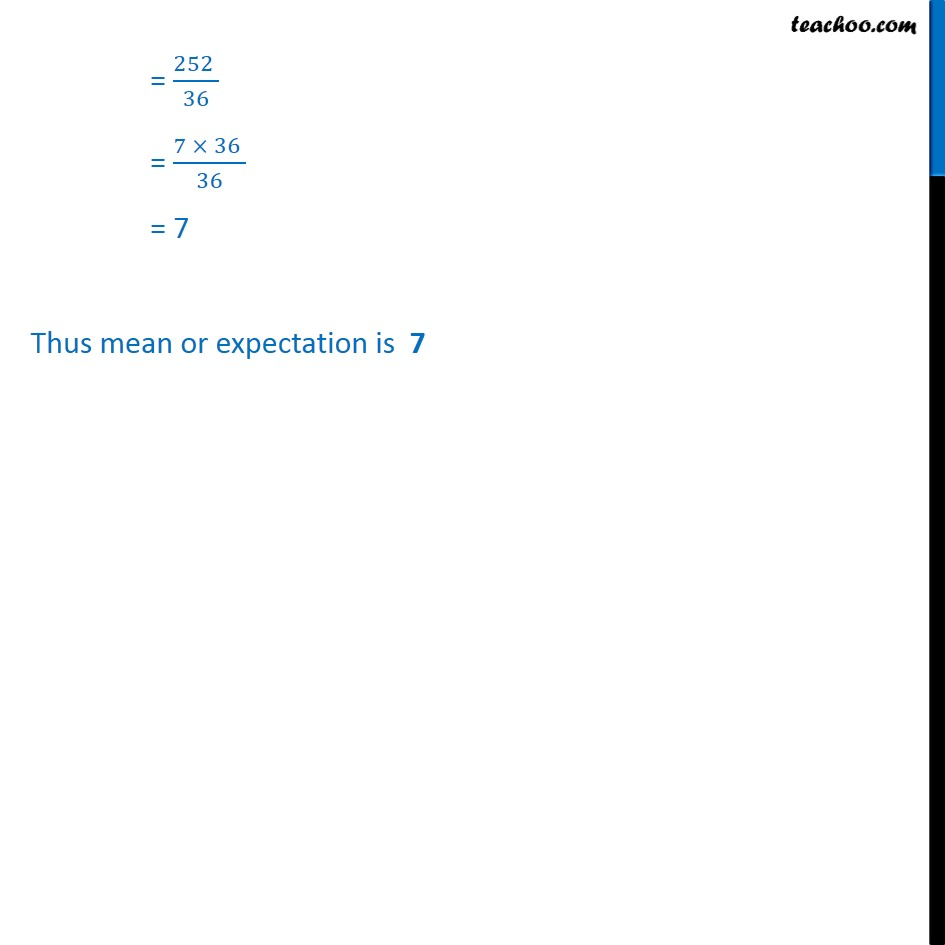Mean or Expectation of random variable

Chapter 13 Class 12 Probability (Term 2)
Concept wise### Transcript

Example 27 Let a pair of dice be thrown and the random variable X be the sum of the numbers that appear on the two dice. Find the mean or expectation of X. Since pair of die are thrown, Here, X = Sum of numbers on two die So, X can be 2, 3, 4, 5, 6, 7, 8, 9, 10, 11, 12 Thus, Probability distribution is Now, We need to find Mean or Expectation of X Mean or Expectation of X = = E(X) = = = 2 1 36 +3 2 36 +4 3 36 +5 4 36 +6 5 36 +7 6 36 +8 5 36 +9 4 36 +10 3 36 +11 2 36 +12 1 36 = 2 + 6 + 12 + 20 + 30 + 42 + 40 + 36 + 30 + 22 + 12 36 = 252 36 = 7 36 36 = 7 Thus mean or expectation is 7